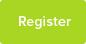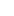# Tutor Hunt Questions

I performed an experiment using egg shells (calcium carbonate) to represent teeth and reacted the calcium carbonate with acids. When measuring the rate of reaction citric acid had a higher rate of reaction and from my research i saw that citric acid forms a more stable compound with calcium hence it chelates the calcium more than ethanoic acid.

In the mouth this would remove calcium ions from
Ca10(PO4)6(OH)2(s) + 2H+(aq) ⇌ 3Ca3(PO4)2(s) + Ca2+(aq) +2H2O(l)
that equation hence the position of equilibrium shifts to the right so more tooth decay.

But with egg shells:
CaCO3(s)+ 2H+ (aq) __> Ca+2(aq) +CO2(g)+H2O(l)

there is no equilibrium so if more calcium ions are chelated why does this increase the rate of reaction?
3 years ago